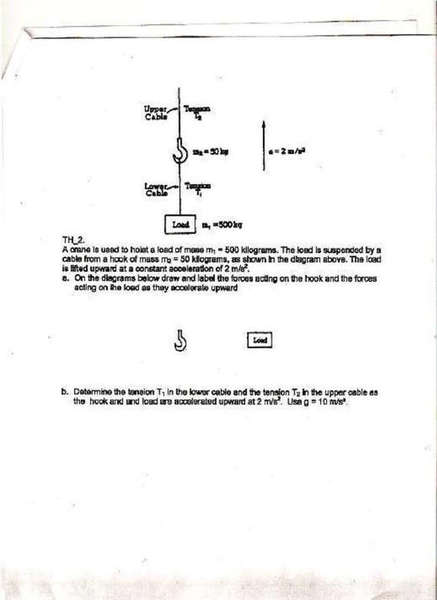# 1982 Physics Problem B

• NavitaLaVida
In summary, the hook and the load are lifted upward at a constant acceleration of 2 m/s^2. The tension in the lower cable is T1 and the tension in the upper cable is T2.

#### NavitaLaVida

[SOLVED] 1982 Physics Problem B

A crane is used to hoist a load of mass m1=500 kilograms. The Load is suspended by a cable from a hook of mass m2=50 kilograms, as shown in the diagram above. The load is lifted upward at a constant acceleration of 2 m/s^2.

b)Determine the Tension T1 in the lower cable and the tension T2 in the upper cable as the hook and load are accelerated upward at 2 m/s^2. Use g=10 m/s^2.

FYI: this is the 1982 Question B from an AP Test. So if you can find a link that has an explanation, that would be great. Or, you can solve it that would also be wonderful! thank you soo much

It'd be nice to see the diagram as,it may show some information not stated and also,you can take a stab at it and we can try to help you out with it.

i don't have a scanner, so I'm sorry but i don't know how i can show you the diagram. i tried to do this problem, i really need help

erm i sent a pic to you
let me know if you can help

hey rock freak
i figured the second part
it's the first part I'm having trouble with

What answers did you get for the 2nd part?

EDIT: well to do the 2nd part,you'd need to know how the forces are acting. Unless you just guessed. Did you?Last edited:
for the second part i used

T1= M1 (g+a)

T2= (M1+M2)(g+a)

it's the first part that I'm not sure about
i get that the force of gravity points down
but what other forces are on it?

NavitaLaVida said:
it's the first part that I'm not sure about
i get that the force of gravity points down
but what other forces are on it?
Tension forces.

NavitaLaVida said:
it's the first part that I'm not sure about
i get that the force of gravity points down
but what other forces are on it?

The only forces to consider are weight and tension. Weight acts downwards so tension must act upwards.

thanks guys!
much appreciated

maybe you all can help me with the resistor problem?